## Technical Articles

Tech Articles from your friends at Oracle and the developer community.

# Understanding, Building and Using Neural Network Machine Learning Models using Oracle 18c

Oracle 18c Database brings prominent new machine learning algorithms, including Neural Networks and Random Forests. While many articles are available on machine learning, most of them concentrate on how to build a model. Very few talk about how to use these new algorithms in your applications to score or label new data. This article will explain how Neural Networks work, how to build a Neural Network in Oracle Database, and how to use the model to score or label new data.

### What are Neural Networks?

Over the past couple of years, Neural Networks have attracted a lot of attention thanks to their ability to efficiently find patterns in data—traditional transactional data, as well as images, sound, streaming data, etc. But for some implementations, Neural Networks can require a lot of additional computing resources due to the complexity of the many hidden layers within the network. Figure 1 gives a very simple representation of a Neural Network with one hidden layer. All the inputs are connected to a neuron in the hidden layer (red circles). A neuron takes a set of numeric values as input and maps them to a single output value. (A neuron is a simple multi-input linear regression function, where the output is passed through an activation function.) Two common activation functions are logistic and tanh functions. There are many others, including logistic sigmoid function, arctan function, bipolar sigmoid function, etc.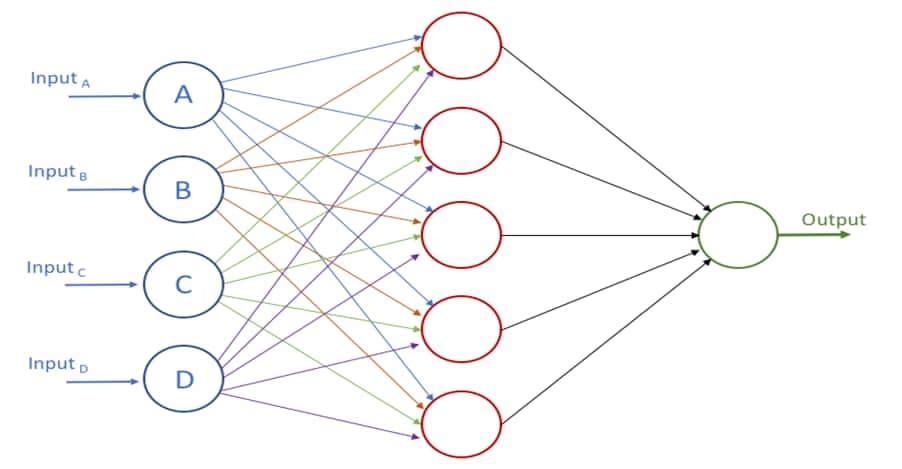Figure 1 – A Simple Neural Network

Figure 1 illustrates a simple Neural Network with one hidden layer. But if additional hidden layers are added, as in Figure 2, the complexity of the computations involved can increase dramatically. The addition of more hidden layers means the Neural Network has additional work to do and additional time is needed to find complex patterns hidden in the data. This gives us machine learning models with greater predictive accuracy compared to more traditional machine learning algorithms. Because there is a computational cost in order to achieve this, you must determine if that additional cost is necessary for your particular problem.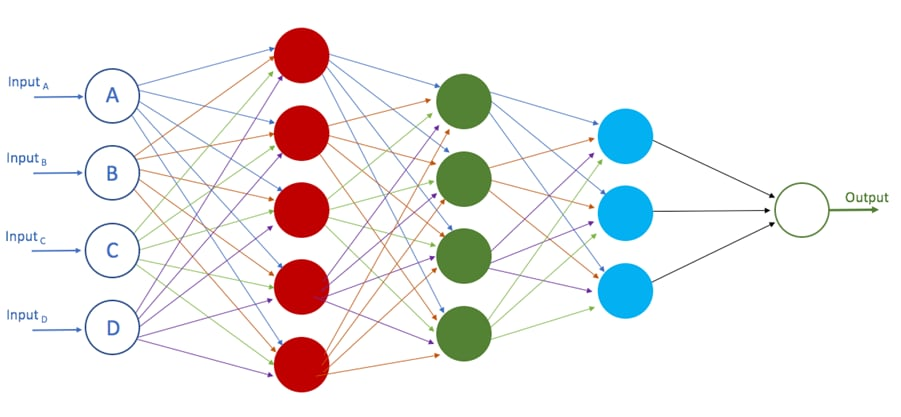Figure 2 – Deep Learning Neural Network

Figure 2 gives an example of a feed-forward Neural Network. With a back-propagation Neural Network, the calculated error rates are fed back to the hidden layers, and the processing at each neuron is updated and the weights rules adjusted to reduce the error rate. This supervised learning method requires a labelled data set. The training of the Neural Network model starts by assuming random weights to each of the connections in the network. The algorithm then iteratively updates the weights in the network by showing training data to the network and updating the network weights until the network is optimized, in a generalized way to avoid overfitting.

This complex and multi-layered approach to building machine learning models gives us the term “deep learning.” Deep learning networks are simply neural networks that have multiple layers of hidden units; in other words, they are deep in terms of the number of hidden layers they have, as illustrated in Figure 2.

Although we commonly read articles that explain how Neural Networks can be applied to image processing, speech recognition, facial recognition, advanced gaming and AI, among many other application areas, Neural Networks are regularly used with regression and classification type of problems. Regression problems look to make predictions based on some numerical value (e.g., estimating the value of a company’s share price on a stock exchange, determining the valuation of a property, acidity levels of wine, etc.), whereas classification is used to predict a defined value. The simplest and most common version of this is binary classification where you want to predict one of two possible outcomes (e.g., if a customer is going to churn or not). An extended version of binary classification is called “multi-class classification,” which is when there are more than two possible outcomes. You can have N number of possible outcomes—but in most scenarios you will have less than 10.

### Neural Networks in Oracle 18c

Oracle has had machine learning algorithms built into the database since Oracle 8i. With each subsequent release new algorithms have been added. These are in addition to the recoding of these algorithms to allow for greater scalability and significant improvement in speed. For example, with a mid-range database server you can now process hundreds of millions of records in seconds. The in-database machine learning algorithms are part of the Advanced Analytics option, which has two components: the in-database machine learning algorithms that can be accessed using SQL, and the ability to embed and run R code inside the database (accessible using R code or SQL). Yes—you can have SQL calling R code.

Neural Networks are included in the release of Oracle 18c database, as part of the Advanced Analytics option. The implementation of the Neural Network incorporates back-propagation and can be used for classification (including binary and multi-class) and regression problems.

In most implementations of Neural Networks algorithms, a lot of work is involved with preparing the data before inputting to the Neural Network. Most of this work revolves around converting data into numerical formats and then normalizing the data.

The in-database Oracle machine learning algorithms have inbuilt Automatic Data Preparation (ADP), a feature that will format, transform and prepare the data—saving you many days of programming.

ADP for Neural Networks replaces missing categorical values with the mode, and missing numerical values with the mean. A Neural Network requires the normalization of numeric input. The algorithm uses z-score normalization. The normalization occurs only for two-dimensional numeric columns (not nested). Normalization places the values of numeric attributes on the same scale and prevents attributes with a large original scale from biasing the solution. A Neural Network scales the numeric values in nested columns by the maximum absolute value seen in the corresponding columns.

### How to Build a Neural Network Model in Oracle 18c

In this section I will provide an example of building a basic Neural Network in Oracle 18c, using the default settings. I will come back to this later to show you how to create a more complex Neural Network by configuring more of the parameter settings.

There are two stages to creating a Neural Network, or any in-database machine learning model. The first part is to define the basic settings. The second part is to run the algorithm based on these settings.

The following code illustrates the creating of a settings table that contains the basic level of parameter settings for the Neural Network. These include telling the in-database machine learning engine to use the Neural Network algorithm, and the second parameter to use the automatic data preparation feature. For the remaining settings and algorithm parameters (see Table 1), Oracle will use the default values, which are based on lots of research and experimental work by Oracle. We will come back to these additional settings later.

``````

-- create the settings table for a Neural Network model
CREATE TABLE demo_Neural_Network_settings
( setting_name  VARCHAR2(30),
setting_value VARCHAR2(4000));

-- insert the settings records for a Neural Network
BEGIN
INSERT INTO demo_neural_network_settings (setting_name, setting_value)
values (dbms_data_mining.algo_name,
dbms_data_mining.algo_neural_network);

INSERT INTO demo_neural_network_settings (setting_name, setting_value)
VALUES (dbms_data_mining.prep_auto, dbms_data_mining.prep_auto_on);
END;

``````
 Setting Name Setting Values Description & Default Value NNET_HIDDEN_LAYERS Value > 0 Defines the number of hidden layers. Default value is 1. NNET_NODES_PER_LAYER Value > 0 Defines the number of nodes per layer. Different layers can have different number of nodes. Default number of nodes is equal to number of input attributes or 50 if the number of attributes > 50. NNET_ACTIVATIONS NNET_ACTIVATIONS_LOG_SIG NNET_ACTIVATIONS_LINEAR NNET_ACTIVATIONS_TANH NNET_ACTIVATIONS_ARCTAN NNET_ACTIVATIONS_BIPOLAR_SIG Activation function for hidden layers. Default value is NNET_ACTIVATIONS_LOG_SIG. NNET_WEIGHT_LOWER_BOUND Number Lower bound of region where weights are randomly initialized. Default is sqrt(6/l_nodes+r_notes)). NNET_WEIGHT_UPPER_BOUND Number Upper bound of region where weights are initialized. Default value is sqrt(6/(l_nodes+r_nodes)) NNET_ITERATIONS Value > 0 The maximum number of iterations. Default value is 200. NNET_TOLERANCE 0 < value < 1 Defines the convergence tolerance setting. Default value is 0.000001. NNET_REGULARIZER NNET_REFULARIZER_NONE NNET_REGULARIZER_L2 NNET_REGULARIZER_HELDASIDE Regularization setting for Neural Network. If training rows > 50,000, the default is NNET_REGULARIZER_HELDASIDE. If <= 50,000, the default is NNET_REGULARIZER_NONE. NNET_HELDASIDE_RATIO 0 <= value <= 1 Defines the held aside ratio. Default value is 0.25. NNET_HELDASIDE_MAX_FAIL Numeric >= 1 With NNET_REGULARIZER_HELDASIDE, the training process is stopped early if the network performance on the validation data fails to improve or remains the same for NNET_HELDASIDE_MAX_FAIL. Default value is 6. NNET_REG_LAMBDA Numeric >= 0 Defines the L2 regularization parameter lambda. Cannot be set at same times as NNET_REGULARIZER_HELDASIZE Default value is 1.

Once the settings have been defined for the Neural Network, the next step is to run the algorithm and generate the model. The function CREATE_MODEL is used to create the machine learning model. This function is part of the DBMS_DATA_MINGING PL/SQL package. The function can be used to create a model with all the in-database machine learning algorithms.

``````

BEGIN
DBMS_DATA_MINING.CREATE_MODEL(
model_name          => 'DEMO_NEURAL_NETWORK_MODEL',
mining_function     => dbms_data_mining.classification,
data_table_name     => 'mining_data_build_v',
case_id_column_name => 'cust_id',
target_column_name  => 'affinity_card',
settings_table_name => 'demo_neural_network_settings');
END;

``````

Table 2 gives a description of the parameters for the CREATE_MODEL function

CREATE_MODEL Parameter Descriptions

 Parameter Name Description MODEL_NAME The name or label of the model. This will be used to identify the model in the database. MINING_FUNCTION The type of problem to be solved. For Neural Networks this can be ‘classification’ or ‘regression’. DATA_TABLE_NAME The name of the table or view that contains the data to be used to build the model. CASE_ID_COLUMN_NAME The attribute that contains the case id for the table. The case id is the unique identifier for each record. If your data set contains a composite identifier, then you will need to create a new attribute that contains a unique number. TARGET_COLUMN_NAME Name of the attribute that contains the target variable to direct the algorithm for classification or regression prediction. SETTINGS_TABLE_NAME The name of the table that contains the additional parameter settings for the algorithm being used.

After the CREATE_MODEL function has completed, the Neural Network model will be created and defined in the database. The next section looks at how you can explore the various properties of the model.

Sample Data Set for the Article

The sample data set being used is based on the Sales History (SH) schema. If you are familiar with the Oracle Data Miner GUI tool, part of SQL Developer, a number of sample data sets and database views are created when you go to use this tool. Some of these views are based on the SH schema and include:

- MINING_DATA_BUILD_V
- MINING_DATA_TEST_V
- MINING_DATA_APPLY_V

### Exploring the Neural Network Model

There are a number of different data dictionary views to allow you to examine the machine learning models. In addition to these general views, there are also some model-specific views that are new in Oracle 18c. Details of these will be given later in this section.

The general data dictionary views consist of:

``````
USER_MINING_MODELS – view high level model detail
USER_MINING_MODEL_SETTINGS – view all parameter settings including defaults
USER_MINING_MODEL_ATTRIBUTES – view attributes used by model

``````

The following examples will illustrate the querying of these and the type of information contained in them. The first view to examine is USER_MINING_MODEL. This view will list all the in-database machine learning model in your schema.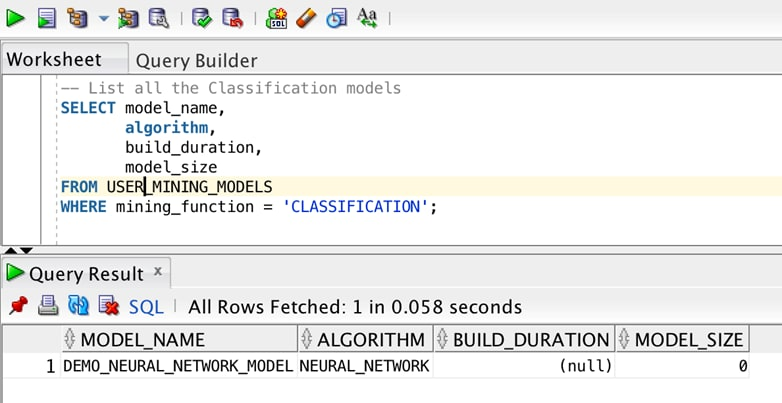Figure 3

When you want to examine in detail all the parameter settings used to create the model, you can use the USER_MINING_MODEL_SETTINGS. This will list the parameters you set up and configured in the settings table (see example above); for those parameters not included in the settings table, the algorithm will use the default settings, as defined in Table 1. This view gives you all of this information in one place.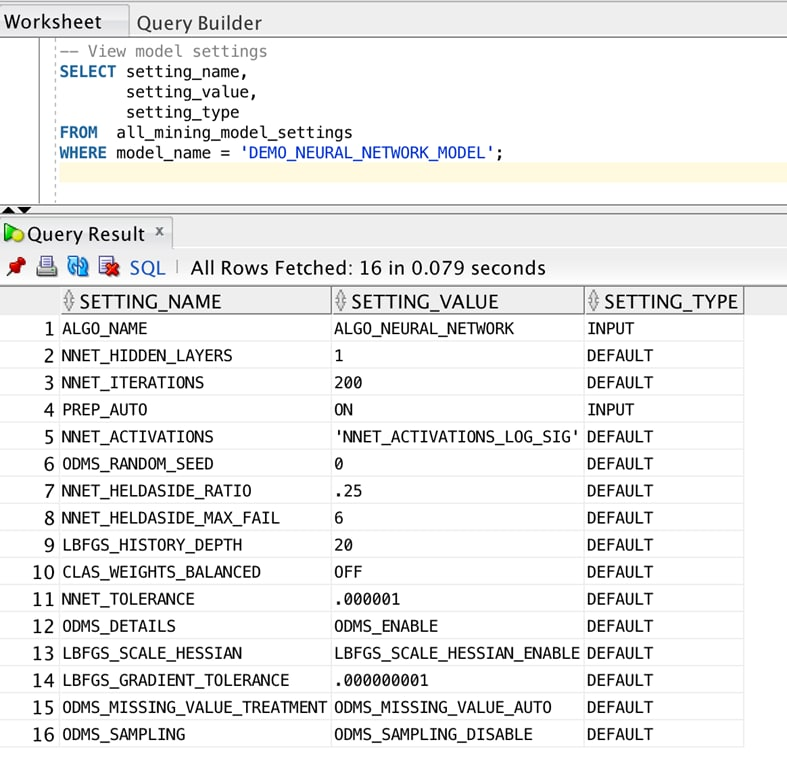Figure 4

When using Oracle 12c Database, or an earlier version, you could use the function GET_MODEL_DETAILS to extract certain information and details of the models. These functions have been deprecated in Oracle 18c. Instead, additional data dictionary views are created. Each type of model (Neural Networks, Decision Trees, Support Vector Machines, etc.) will have their own properties and hence will present slightly different details in each of the data dictionary views. These can be identified with the prefix of DM\$XX, where XX will be one of VA, VC, VG, VN, VS, VT and VW, followed by the name of the machine learning model.

The data dictionary views created for the Neural Network created in this article include:

``````
DM\$VADEMO_NEURAL_NETWORK_MODEL – weights of the neurons
DM\$VCDEMO_NEURAL_NETWORK_MODEL – scoring cost matrix
DM\$VGDEMO_NEURAL_NETWORK_MODEL – global statistics
DM\$VNDEMO_NEURAL_NETWORK_MODEL – normalization parameters
DM\$VSDEMO_NEURAL_NETWORK_MODEL – computed settings
DM\$VTDEMO_NEURAL_NETWORK_MODEL – describes the target distribution
DM\$VWDEMO_NEURAL_NETWORK_MODEL – alerts during model build
``````

If you inspect the contents of `DM\$VNDEMO_NEURAL_NETWORK_MODEL` you will get to see the outputs from the Automatic Data Preparation (ADP) that was set earlier.

### How to use the Neural Network Model

In the previous section I showed how easy it was to create a Neural Network machine- learning model in an Oracle 18c Database. The next thing we will look at is how you can use the model to label new data. This is something that will typically be required in your front-end applications, your web-based application or in your back-end or batch applications and processes. Any programming language or framework that can call SQL can now run the in-database Neural Network model by using a simple SQL command.

The following examples illustrates how simple the SQL is for using the Neural Network model. We can spend a lot of time going through many different possibilities of using the model, but the next two examples illustrate the simplicity involved.

SQL has a number of analytics functions that are associated with calling an in-database machine learning model. The following examples will illustrate the two most commonly used of these SQL functions, which tell you the predicted value and the prediction probability or the strength of the prediction by the model (a confidence score).

Our first example uses the PREDICTION and PREDICTION_PROBABILITY SQL function. The PREDICTION function returns the predicted value from the model. It takes as input the name of the machine learning model to use and the list of attributes to input to the model. In our example I have used the * (star) to indicate to the model to use all available attributes from the selected table or view.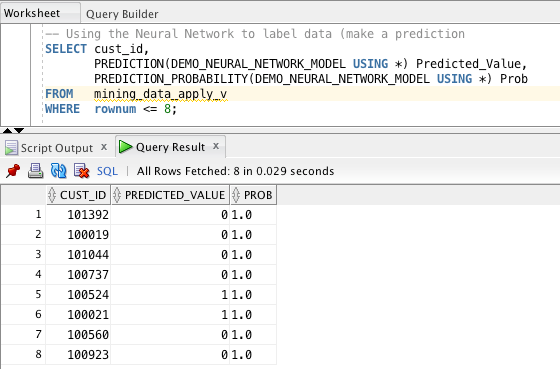Figure 5

The PREDICTION_PROBABILTY function returns a confidence score, on a zero to one scale, of how strong a prediction the model has made. Getting a value of 1 will indicate that the model is 100% sure the prediction is correct. Getting a value in the range 0.75 to 1 is generally a strong prediction.

We need to remember that the model is making a prediction. If we have a good model, it should be correctly predicting a large percentage of values correctly. A model will never be 100% accurate as a machine learning model is a generalized representation of the data used to train the model. When the model is used against new, previously unseen data, it will make a prediction based on information in the model. Sometimes it will make an incorrect prediction. As you gather more and more data, this data can be added into the training data set, thereby increasing the potential accuracy of future versions of the model

You can see how simple this query is and how easily it could be integrated into our applications developed in other languages.

But the previous example is based on data stored in a database table—and not all data lives in a database table (e.g., data created in an application that hasn’t yet been persisted to the database). This is common in very dynamic applications. The following example illustrates how you can still use the Neural Network model stored in the data to make a prediction based on data in your application. This is an example of doing “what-if” analysis. In our application, some of this data could be represented as sliders and other objects. As these sliders are adjusted, the application needs to dynamically recalculate the prediction from the model in real-time and to make the necessary updates to the application.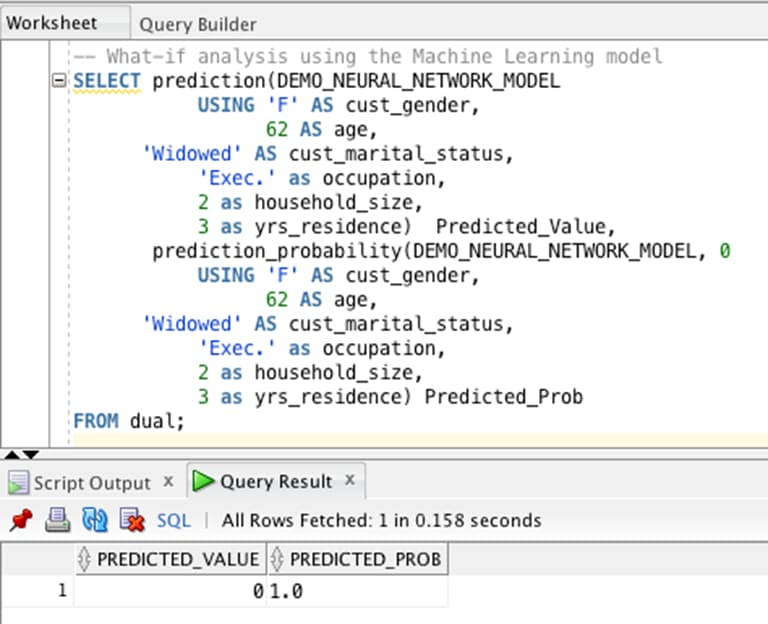Figure 6

### Let’s go Deep!  Creating a Deep Learning Neural Network

The examples shown above create a very simple (i.e., with one hidden layer) neural network model. All input attributes are mapped to all the nodes in the one hidden layer and are used to make a prediction.

Typically, the term deep learning is used in conjunction with neural networks, but all too often there can be some confusion about what “deep learning” actually means. It is a term commonly used when using neural networks on image files. To keep things simple, deep learning is when multiple hidden layers are used in the neural network model build process. Earlier, I described how the input attributes are used, along with different weightings and an activation function, to define the node in a hidden layer. This is complicated set of mathematics that produces an output. With deep learning, this complicated set of mathematics is then used as input to another hidden layer. This output from one node is combined with similar outputs from other nodes. This is illustrated in Figure 2 and the hidden layers colored in green and blue. The more hidden layers and nodes at each layer, the more complex the process, but also the greater the ability to find deep hidden patterns in the data, patterns found by combining different attributes in different ways. For example, when the attributes of a person’s height and weight are combined using a particular formula to form a BMI value (Body Mass Index), we get better, more meaningful, insight into a person’s body make up and risk factors.

There are a couple of options for setting up different hidden layers and nodes in each layer, for the neural network algorithm in Oracle 18c. The first is to define how many hidden layers to use. The algorithm will then work out the number of nodes for each layer, but this may match the number of input variables at each hidden layer. An alternative is to define the number of nodes to use at each hidden layer. This is set up as a parameter in a settings table. The following is an extended version of the previous settings table with an additional parameter of NNET_NODES_PER_LAYER. The number of hidden layers does not need to be defined when using this parameter. The following example defines for the neural network to have three hidden layers with ten nodes at the first hidden layer, six nodes at the second hidden layer and four nodes at the third hidden layer.

``````
CREATE TABLE demo_Neural_Network_settings_2
( setting_name  VARCHAR2(30),
setting_value VARCHAR2(4000));

-- insert the settings records for a Neural Network
BEGIN
INSERT INTO demo_neural_network_settings_2 (setting_name, setting_value)
values (dbms_data_mining.algo_name, dbms_data_mining.algo_neural_network);

INSERT INTO demo_neural_network_settings_2 (setting_name, setting_value)
VALUES (dbms_data_mining.prep_auto, dbms_data_mining.prep_auto_on);

INSERT INTO demo_neural_network_settings_2 (setting_name, setting_value)
VALUES (dbms_data_mining.nnet_nodes_per_layer, '10,6,4');
END;
``````

The big question is: Do we get a better model when using the additional hidden layers (deep learning)? The answer is “it depends.” It depends on the number of layers and nodes at each layer. There are many approaches to working out the appropriate number for each, and there will be a bit of experimental work in trying to find the optimal amount. It will require evaluating the performance of each iteration of the model settings. This can be easily scripted and is dependent on having a holdout data set to evaluate the model. The data set being used in this article also contains a separate data set for testing the model. This is called MINING_DATA_TEST_V and contains a different subset of the SH schema to what is used in MINING_DATA_BUILD_V view.

The first step is to create a view that uses the model to label the test data. The second step is to compute the confusion matrix for the model based on the predicted values compared to the actual values. This computes how many predictions it correctly and incorrectly predicted.

``````
CREATE OR REPLACE VIEW demo_nnet_test_results_2
AS
SELECT cust_id,
prediction(DEMO_NEURAL_NETWORK_MODEL_2 USING *)  predicted_value,
prediction_probability(DEMO_NEURAL_NETWORK_MODEL_2 USING *) probability
FROM   mining_data_test_v;

DECLARE
v_accuracy NUMBER;
BEGIN
DBMS_DATA_MINING.COMPUTE_CONFUSION_MATRIX (
accuracy 			    => v_accuracy,
apply_result_table_name	    => 'demo_nnet_test_results_2',
target_table_name 	    => 'mining_data_test_v',
case_id_column_name 	    => 'cust_id',
target_column_name 	    => 'affinity_card',
confusion_matrix_table_name => 'demo_nnet_confusion_matrix_2',
score_column_name 	    => 'PREDICTED_VALUE',
score_criterion_column_name => 'PROBABILITY',
cost_matrix_table_name	    => null,
apply_result_schema_name    => null,
target_schema_name 	    => null,
cost_matrix_schema_name     => null,
score_criterion_type 	    => 'PROBABILITY');
DBMS_OUTPUT.PUT_LINE('**** MODEL ACCURACY ****: ' || ROUND(v_accuracy,4));
END;
``````

When this evaluation approach was used for the first neural network model (with one hidden layer), a model accuracy of 78% was achieved—3% higher than the other in-database machine learning models. This is good and shows how powerful neural networks are compared to more traditional machine learning algorithms. When the second neural network model was evaluated, an accuracy of 81% was achieved. That is now a 6% better performance compared to the next best machine learning model. This is a major achievement. With time and patience, the number of hidden layers and number of nodes at each layer could be fine-tuned further to gain a better result.

### Summary

Neural Networks are a powerful machine learning algorithm, allowing you to create complex and deep learning neural network models to find hidden patterns in your data sets. Neural Networks are available with Oracle 18c and can be easily built and used to make predictions using a few simple SQL commands. These can be easily REST enabled, making it simple to incorporate into any other programming language or framework.

Brendan Tierney, Oracle Groundbreaker Ambassador & Oracle ACE Director, is an independent consultant (Oralytics) and lectures on data science, databases, and Big Data at the Dublin Institute of Technology/Dublin Technological University. He has 25+ years of experience working in the areas of data mining, data science, Big Data, and data warehousing. Brendan is a recognized Data Science and Big Data expert and has worked on projects in Ireland, the UK, Belgium, Holland, Norway, Spain, Canada, and the U.S. He is active in the Oracle User Group community, where he is one of the leaders for the OUG in Ireland and is a Member Advocate at Board of Director level with the UKOUG. Brendan has also been editor of the UKOUG Oracle Scene magazine and is a regular speaker at conferences around the world. He is an active blogger and also writes articles for OTN, Oracle Scene, IOUG SELECT Journal, ODTUG Technical Journal, and ToadWorld. He is also on the board of directors for DAMA in Ireland. Brendan has published four books, three with Oracle Press/McGraw-Hill (Predictive Analytics Using Oracle Data Miner, Oracle R Enterprise: Harnessing the Power of R in Oracle Database, and Real World SQL and PL/SQL: Advice from the Experts) and one with MIT Press (Essentials of Data Science). These books are available on Amazon, in print, ebook and audio book formats.

Latest content

Explore and discover our latest tutorials

## Serverless functions

Serverless functions are part of an evolution in cloud computing that has helped free organizations from many of the constraints of managing infrastructure and resources.

## What is a blockchain?

In broad terms, a blockchain is an immutable transaction ledger, maintained within a distributed peer-to-peer (p2p) network of nodes. In essence, blockchains serve as a decentralized way to store information.

## OCI CLI

The CLI is a small-footprint tool that you can use on its own or with the Console to complete Oracle Cloud Infrastructure tasks. The CLI provides the same core functionality as the Console, plus additional commands.